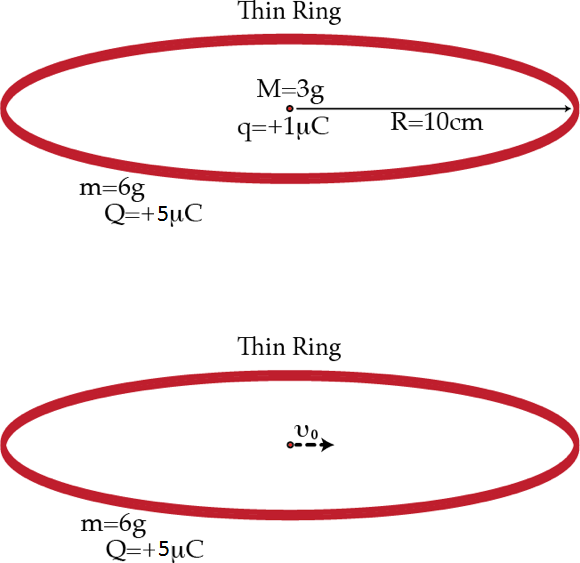# Interaction between a point charge and a ring

A point charge $q=+1~\mu\mbox{C}$ with mass $M=3~\mbox{g}$ is placed at the center of a thin ring of radius $R=10~\mbox{cm}$, mass $m=6~\mbox{g}$, and charge $Q=5 \mu\mbox{C}$. Initially, the system is at rest in equilibrium. Then, the point charge is given a push so that it acquires a small velocity $\vec{v}_{0}$ in the plane of the ring. Determine the minimum time $\tau$ in seconds after which the point charge will be at the center of the ring again. Assume that the charge in the ring is distributed uniformly. The following expansion might be useful: $\frac{1}{\sqrt{1+\frac{x^{2}}{R^{2}}-2 \frac{x}{R}\cos(\theta)}}\approx 1+\cos(\theta) \frac{x}{R}-\frac{1-3 \cos^{2}(\theta)}{2}\frac{x^{2}}{R^{2}}$ for $x \ll R$.Details and assumptions

• $k=\frac{1}{4\pi \epsilon_{0}}= 9\times 10^{9}~\mbox{m/F}.$

• Neglect gravitational forces.

• The ring is free to move.

×

Problem Loading...

Note Loading...

Set Loading...